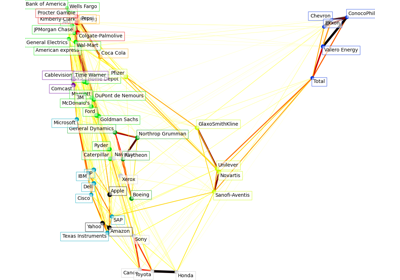# sklearn.cluster.affinity_propagation¶

sklearn.cluster.affinity_propagation(S, *, preference=None, convergence_iter=15, max_iter=200, damping=0.5, copy=True, verbose=False, return_n_iter=False, random_state=None)[source]

Perform Affinity Propagation Clustering of data.

Read more in the User Guide.

Parameters:
Sarray-like of shape (n_samples, n_samples)

Matrix of similarities between points.

preferencearray-like of shape (n_samples,) or float, default=None

Preferences for each point - points with larger values of preferences are more likely to be chosen as exemplars. The number of exemplars, i.e. of clusters, is influenced by the input preferences value. If the preferences are not passed as arguments, they will be set to the median of the input similarities (resulting in a moderate number of clusters). For a smaller amount of clusters, this can be set to the minimum value of the similarities.

convergence_iterint, default=15

Number of iterations with no change in the number of estimated clusters that stops the convergence.

max_iterint, default=200

Maximum number of iterations.

dampingfloat, default=0.5

Damping factor between 0.5 and 1.

copybool, default=True

If copy is False, the affinity matrix is modified inplace by the algorithm, for memory efficiency.

verbosebool, default=False

The verbosity level.

return_n_iterbool, default=False

Whether or not to return the number of iterations.

random_stateint, RandomState instance or None, default=None

Pseudo-random number generator to control the starting state. Use an int for reproducible results across function calls. See the Glossary.

New in version 0.23: this parameter was previously hardcoded as 0.

Returns:
cluster_centers_indicesndarray of shape (n_clusters,)

Index of clusters centers.

labelsndarray of shape (n_samples,)

Cluster labels for each point.

n_iterint

Number of iterations run. Returned only if return_n_iter is set to True.

Notes

For an example, see examples/cluster/plot_affinity_propagation.py.

When the algorithm does not converge, it will still return a arrays of cluster_center_indices and labels if there are any exemplars/clusters, however they may be degenerate and should be used with caution.

When all training samples have equal similarities and equal preferences, the assignment of cluster centers and labels depends on the preference. If the preference is smaller than the similarities, a single cluster center and label 0 for every sample will be returned. Otherwise, every training sample becomes its own cluster center and is assigned a unique label.

References

Brendan J. Frey and Delbert Dueck, “Clustering by Passing Messages Between Data Points”, Science Feb. 2007

## Examples using sklearn.cluster.affinity_propagation¶Visualizing the stock market structure

Visualizing the stock market structure